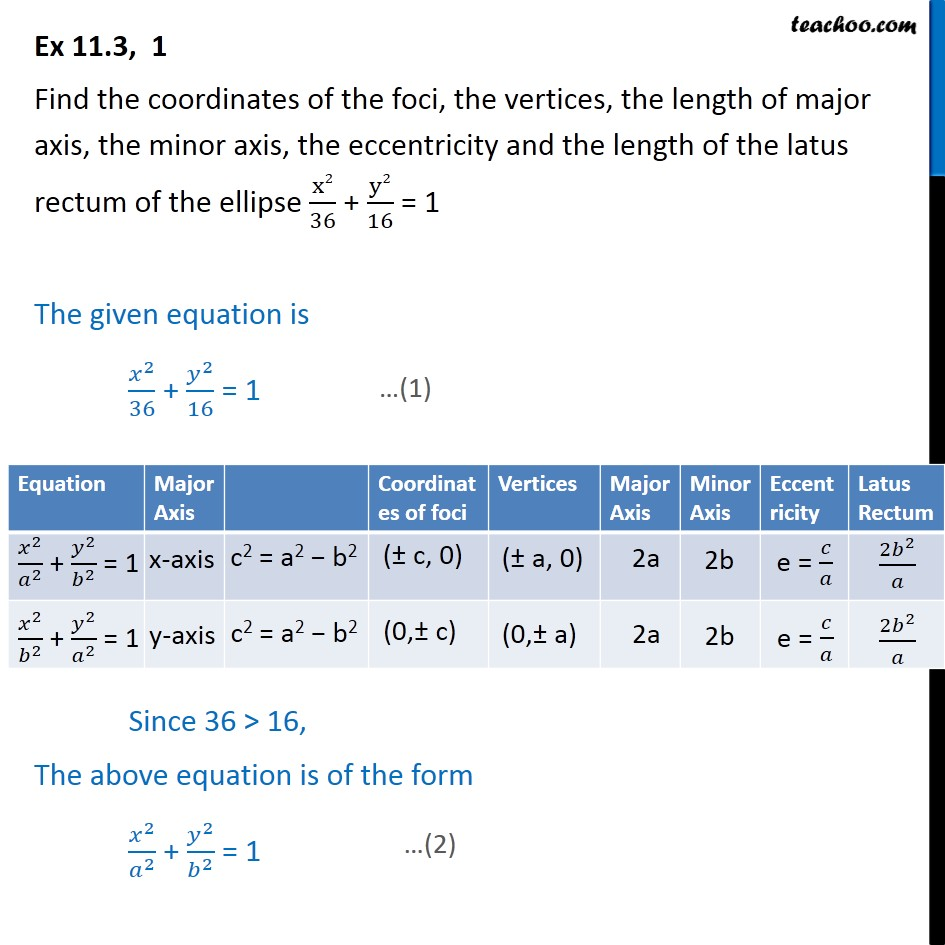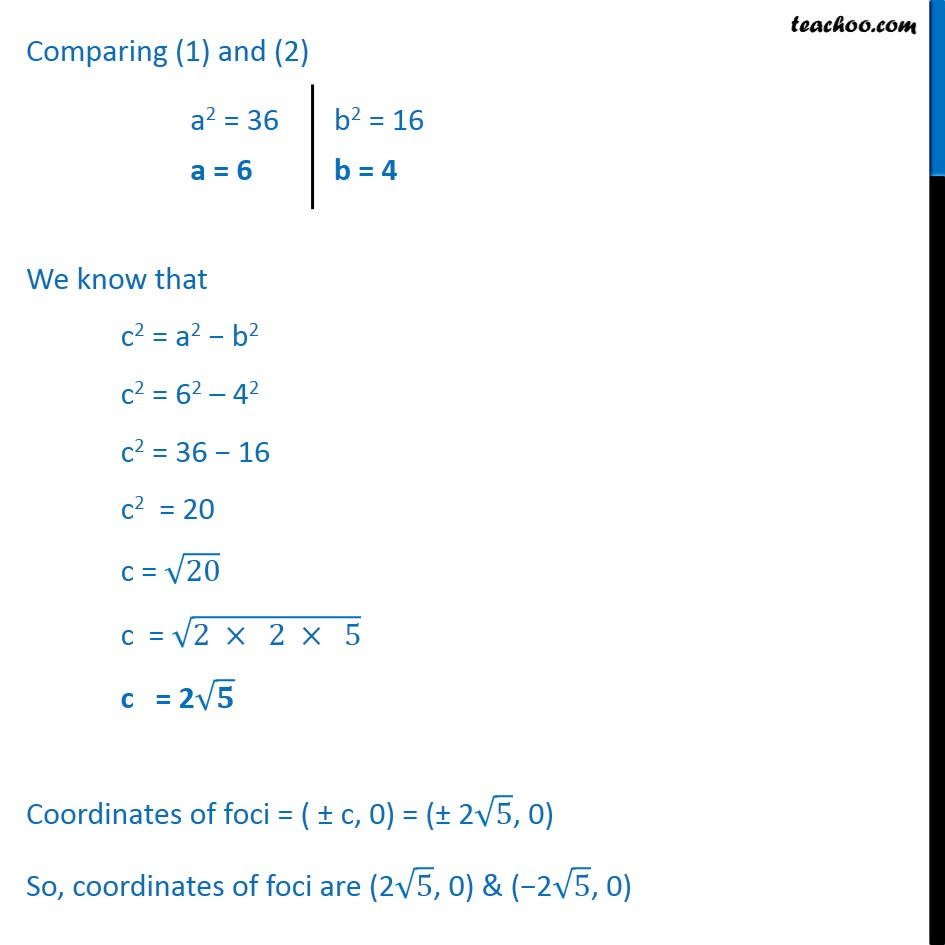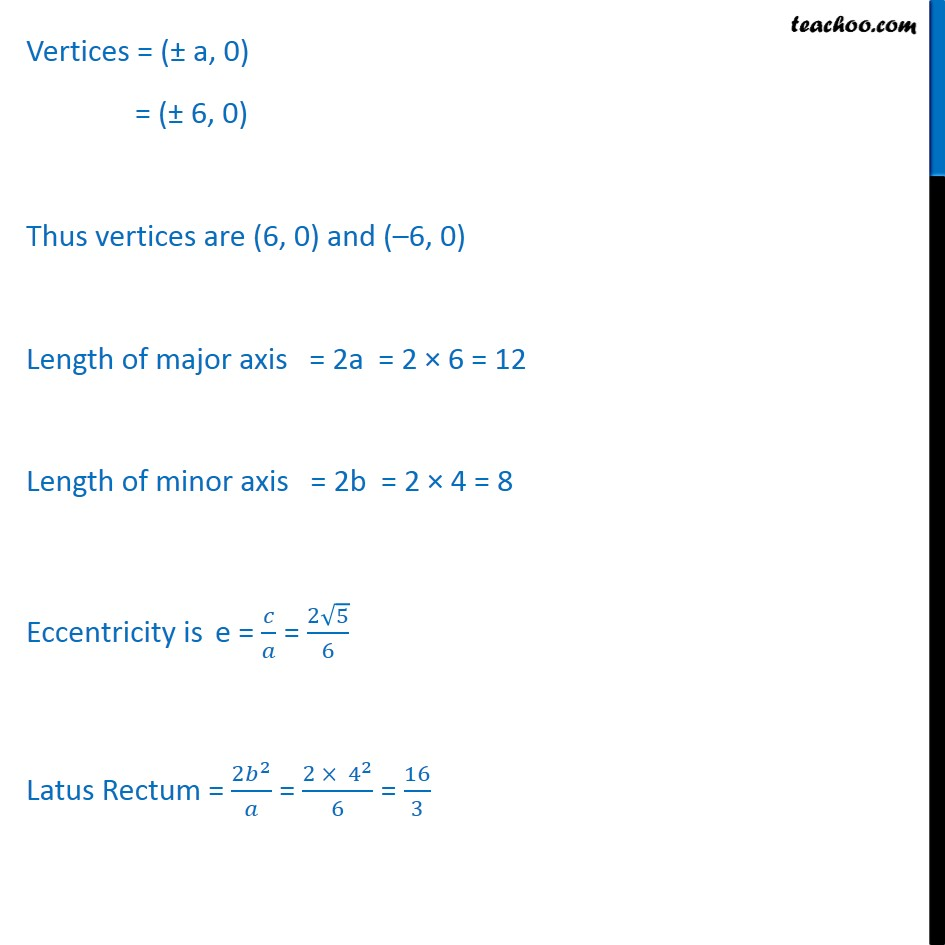Ellipse - Defination

Chapter 10 Class 11 Conic Sections
Concept wiseLearn in your speed, with individual attention - Teachoo Maths 1-on-1 Class

### Transcript

Ex 10.3, 1 Find the coordinates of the foci, the vertices, the length of major axis, the minor axis, the eccentricity and the length of the latus rectum of the ellipse ﷐x2﷮36﷯ + ﷐y2﷮16﷯ = 1 The given equation is ﷐﷐𝑥﷮2﷯﷮36﷯ + ﷐﷐𝑦﷮2﷯﷮16﷯ = 1 Since 36 > 16, The above equation is of the form ﷐﷐𝑥﷮2﷯﷮﷐𝑎﷮2﷯﷯ + ﷐﷐𝑦﷮2﷯﷮﷐𝑏﷮2﷯﷯ = 1 Comparing (1) and (2) We know that c2 = a2 − b2 c2 = 62 – 42 c2 = 36 − 16 c2 = 20 c = ﷐﷮20﷯ c = ﷐﷮2 × 2 × 5﷯ c = 2﷐﷮𝟓﷯ Coordinates of foci = ( ± c, 0) = (± 2﷐﷮5﷯, 0) So, coordinates of foci are (2﷐﷮5﷯, 0) & (−2﷐﷮5﷯, 0) Vertices = (± a, 0) = (± 6, 0) Thus vertices are (6, 0) and (–6, 0) Length of major axis = 2a = 2 × 6 = 12 Length of minor axis = 2b = 2 × 4 = 8 Eccentricity is e = ﷐𝑐﷮𝑎﷯ = ﷐2﷐﷮5﷯﷮6﷯ Latus Rectum = ﷐2﷐𝑏﷮2﷯﷮𝑎﷯ = ﷐2 × ﷐4﷮2﷯﷮6﷯ = ﷐16﷮3﷯